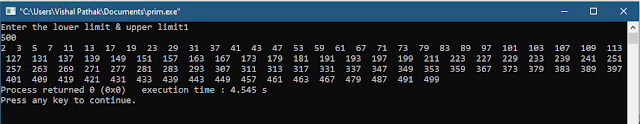### Recents in Beach# Prime Numbers - How to find prime number in c program?

In this tutorial, we are going to know about, how we can find prime numbers when the user enters the range.

for example :

Let's suppose the range is 10 - 100, then we have to find the prime numbers between them.

So, Let get started.

### Program to find Prime Number :

`````` #include<stdio.h>
#include<conio.h>
int main(){
int lower_limit , upper_limit,flag;
printf("Enter the lower limit & upper limit");
scanf("%d%d",&lower_limit,&upper_limit);
for(int i= lower_limit ;i<=upper_limit ; i++){
flag =0;
for(int j =2 ;j<=i/2;j++){
if(i%j==0){
flag ++;
break;
}
}
if(flag==0 && i != 1){
printf("%d " ,i);
}
}
return 0;
}  ``````

Output :Output of prime numbers in a range of 1 - 500

### Explanation :

The program above find prime number between some range entered by user and print's the prime number found between this range.

Currently , We are using Nested for loop for this operation.

In the first part of the loop i,e first for loop , we initialized 'i' with lower limit and compare i< = upper_limit because we have to go from lower limit to upper limit .

For example :

let suppose we have to check prime number between 1 - 100 then we have to start with 1 then we have to move forward like 2 then 3, 4, 5 ......, 100 to judge the number one by one whether they are prime or not.

Moving on to next step we are initializing flag with 0 then second for loop starts.

In second for we initialized 'j' with 2 because from definition of prime number we know :

" Prime Numbers are the number that has only 2 factors: 1 and themselves."

therefore if a number is divisible by 2 is not a prime number.

Moving forward we compare j<=i/2 after this we are dividing 'i' by 'j' to get remainder.

If it is 0 then we are increasing flag value with one and then break (to break second for loop).

When loop breaks we compare flag 0 & i don't equal to 1 if it is true then we print the number.

Why j<=i/2 ? :

Consider an example if we want to know whether 15 is prime or not? , then what we do?

we must divide it from 2 to 7 to check the remainder is zero or not because after 7, dividing 15 with the numbers next 7 we always get remainder greater than 0 then there is an increment in the value of flag leading to a wrong answer.

### Prime Numbers Summary :

So ,this is the complete tutorial to find prime number in c program .

What we conclude from tutorial that we must use nested loop.

The first loop must start from lower limit and end's to upper limit the second loop starts from 2 and end's at half of current position of lower limit.

Then dividing them to get the remainder if remainder is zero then print the number .